# Introduction to solving equations with Variables

### Introduction to solving equations with Variables

#### Lessons

In this lesson, we will learn:

• How to isolate the variable in an equation by using equation models, or by doing the operation to both sides to rearrange equations
• How to understand multiplication and division questions using the phrase “times as many” with the “times as many’ model

Notes:

• A systematic way to solve equations and find unknown variables is to isolate the variable:
• We want the unknown variable by itself on one side of the equal sign (=) and only numbers on the other side so that the operation can be completed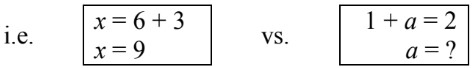• Recall the equation models for the four operations. Equation models can help rearranging parts of an equation to isolate the variable. Any simple equation can be rewritten in 3 ways. Out of the three ways, one version will have the unknown variable by itself.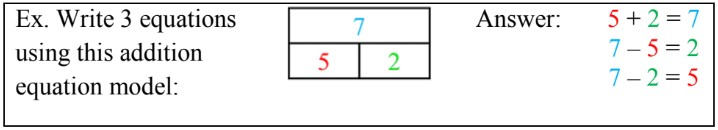• When equation parts are side by side, you will be adding/multiplying; when equation parts are on top of each other, you will be subtracting/dividing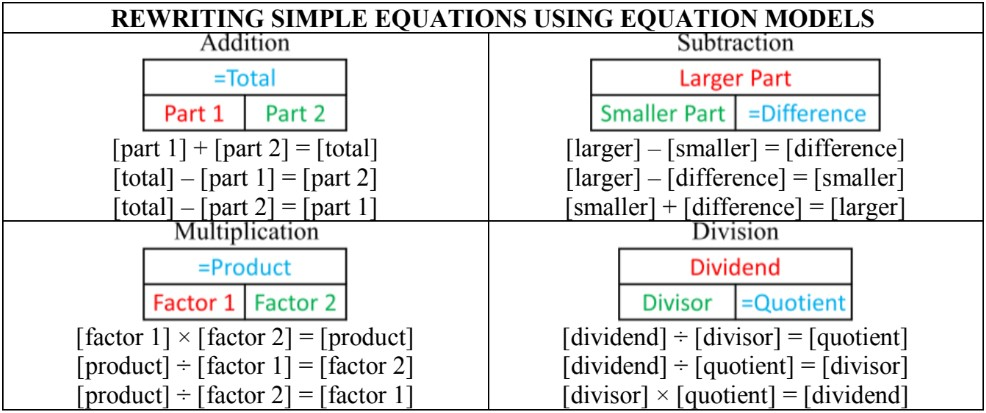• You can also isolate the variable by “doing the opposite
operation to both sides”: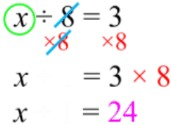• Word problems using the phrase “times as many” may need multiplication or division:
• Use the “times as many” model to decide which operation to use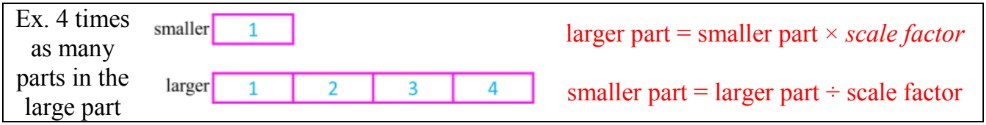• Introduction
Introduction to Introduction to Solving Equations with Variables:
a)
Solving the equation means isolating the variable

b)
Isolate the variable by rewriting a simple equation in three ways

c)
Isolate the variable by doing the opposite operation to both sides of the equation

d)
Solving equations with the "times as many" model

• 1.
Rewriting equations with variables: addition and subtraction
Use the equation table to write 3 different equations. Circle when the unknown variable is by itself and solve.
a)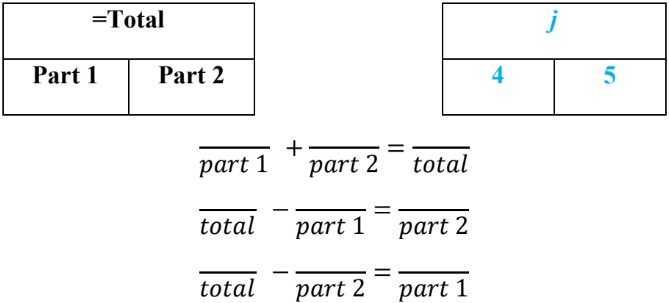b)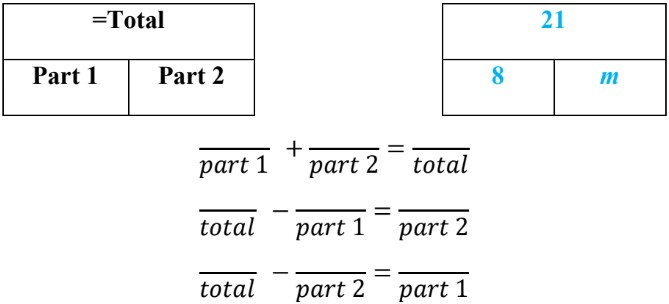c)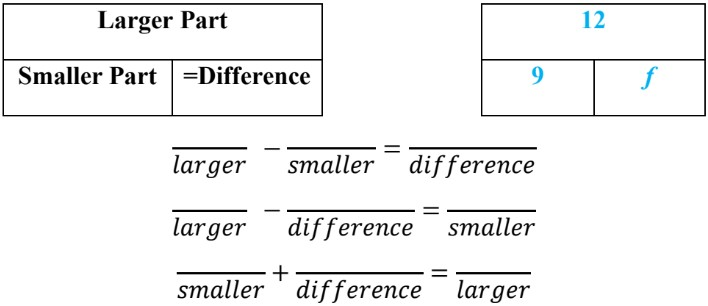d)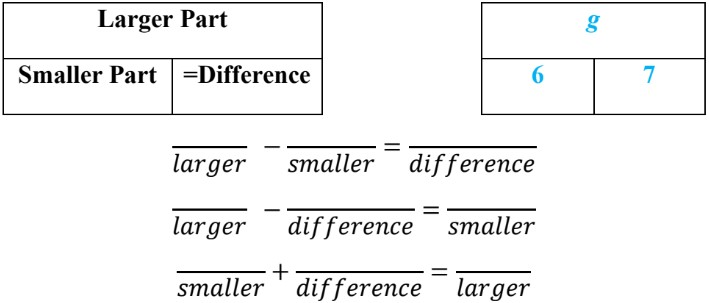• 2.
Rewriting equations with variables: multiplication and division
Use the equation table to write 3 different equations. Circle when the unknown variable is by itself and solve.
a)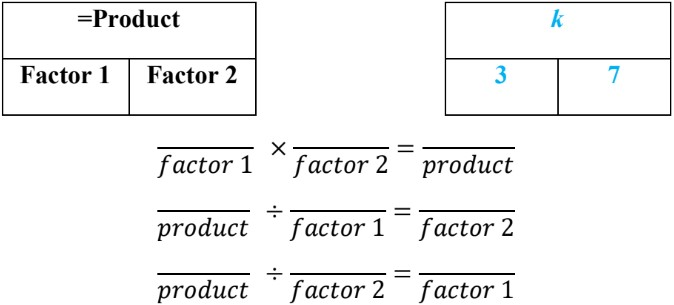b)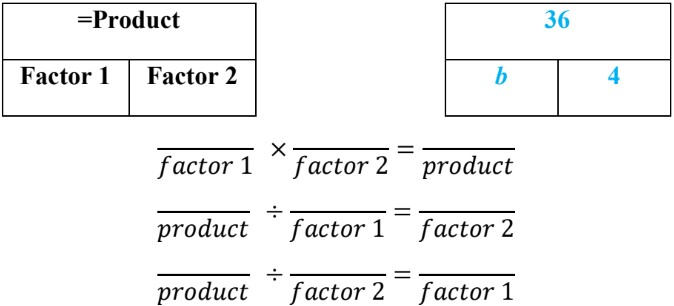c)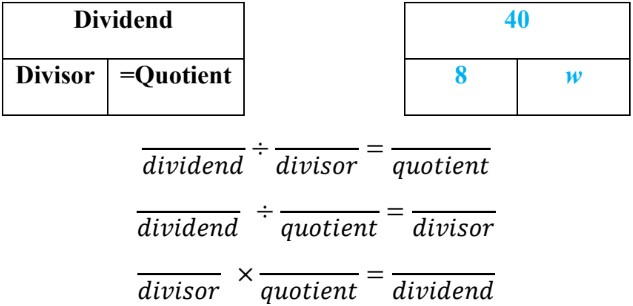d)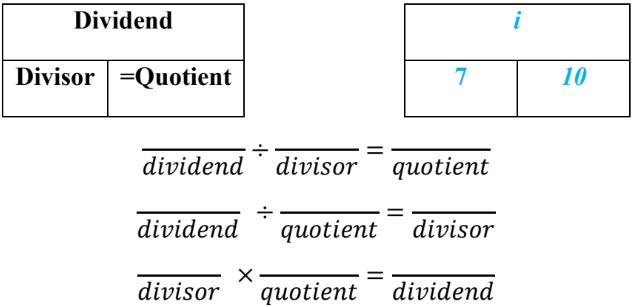• 3.
Solving equations by isolating the variable
Get the unknown variable by itself to solve. Use the opposite operation on both sides.
a)
$n$ + 38 = 62

b)
$q$ - 47 = 51

c)
$d$ × 9 = 54

d)
$z$ ÷ 6 = 8

• 4.
Solving equations: "times as many" models
Label the "times as many" model for the question. Write an equation using the scale factor and solve.
a)
There are 4 times as many ants as there are butterflies. There are 10 butterflies. How many ants are there?b)
There are 3 times as many circles as there are diamonds. There is a total of 8 shapes altogether. How many circles are there?c)
There are 25 pigs on the farm. There are 5 times as many pigs as horses. How many horses are there?d)
There are 6 times as many red cars as blue cars in the mall parking lot. There are 40 more red cars as blue cars in the mall parking lot. How many blue cars are there?• 5.
Solving equations: word problems
Write an equation for the word problem and solve.
a)
There are 5 brown-haired dogs and 7 blonde-haired dogs at the pet adoption center.
1. How many dogs are there in total?

2. Some of the dogs are adopted later that day. If there are only 8 dogs left, how many dogs were adopted?

b)
There are 12 red jelly beans. There are 3 times as many green jelly beans as red jelly beans.
1. How many green jelly beans are there?

2. If there are 2 times as many green jelly beans as yellow jelly beans, how many yellow jelly beans are there?

3. How many jelly beans are there in total?

c)
Alice is 4 years younger than Benji. Benji is twice as old as Cassidy. Donna is six times as old as Cassidy . Donna is 42. How old is Alice?